# PPT on refraction and lenses by pg

2 de Nov de 2012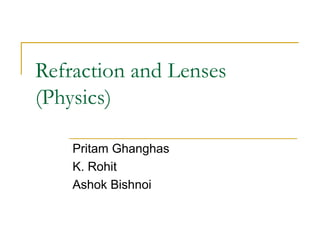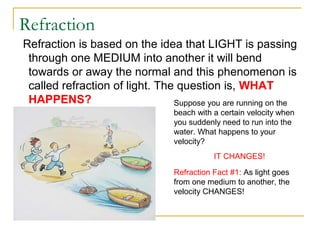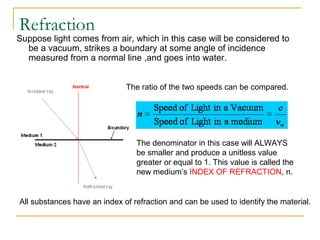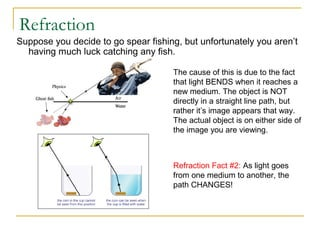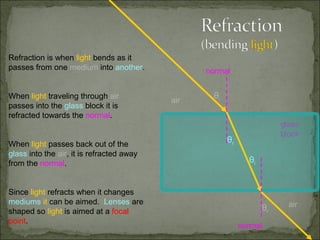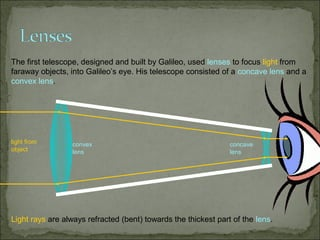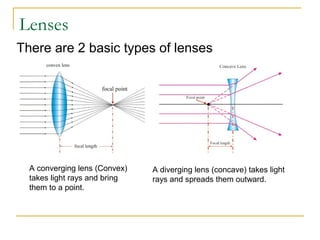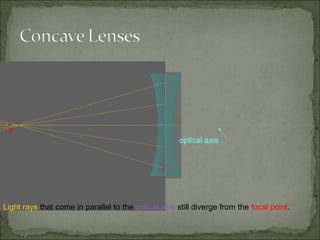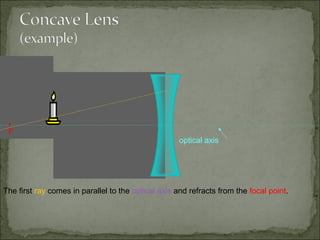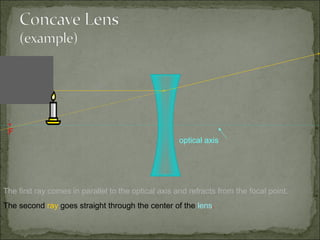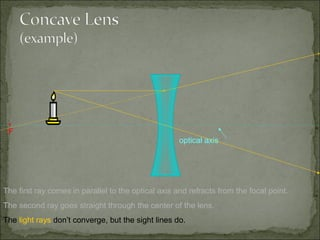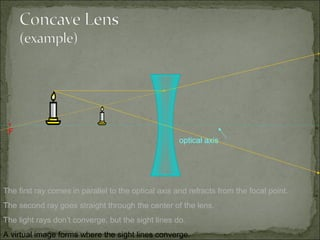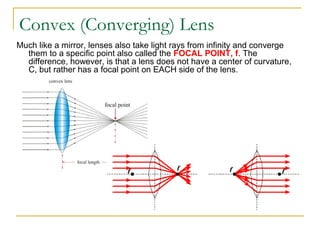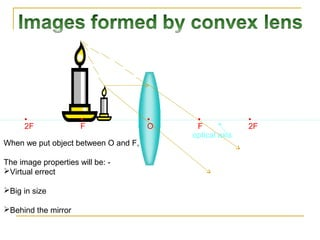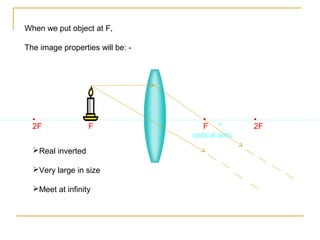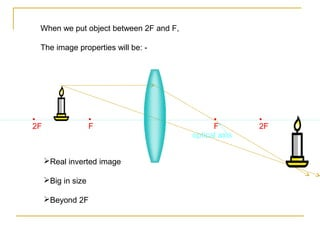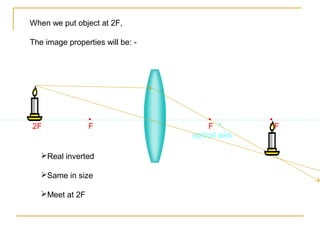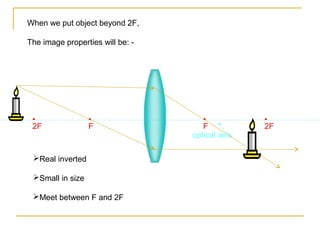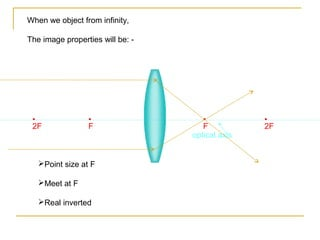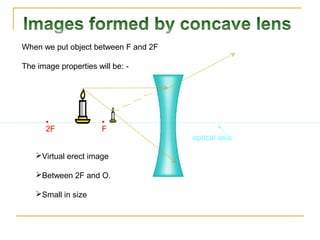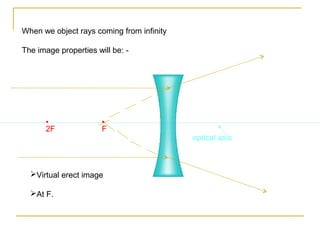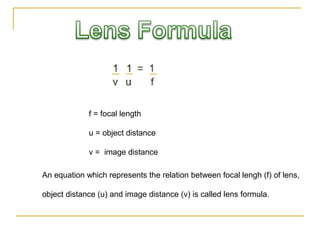1 de 23

### PPT on refraction and lenses by pg

• 1. Refraction and Lenses (Physics) Pritam Ghanghas K. Rohit Ashok Bishnoi
• 2. Refraction Refraction is based on the idea that LIGHT is passing through one MEDIUM into another it will bend towards or away the normal and this phenomenon is called refraction of light. The question is, WHAT HAPPENS? Suppose you are running on the beach with a certain velocity when you suddenly need to run into the water. What happens to your velocity? IT CHANGES! Refraction Fact #1: As light goes from one medium to another, the velocity CHANGES!
• 3. Refraction Suppose light comes from air, which in this case will be considered to be a vacuum, strikes a boundary at some angle of incidence measured from a normal line ,and goes into water. The ratio of the two speeds can be compared. The denominator in this case will ALWAYS be smaller and produce a unitless value greater or equal to 1. This value is called the new medium’s INDEX OF REFRACTION, n. All substances have an index of refraction and can be used to identify the material.
• 4. Refraction Suppose you decide to go spear fishing, but unfortunately you aren’t having much luck catching any fish. The cause of this is due to the fact that light BENDS when it reaches a new medium. The object is NOT directly in a straight line path, but rather it’s image appears that way. The actual object is on either side of the image you are viewing. Refraction Fact #2: As light goes from one medium to another, the path CHANGES!
• 5. Refraction is when light bends as it passes from one medium into another. normal When light traveling through air air θi passes into the glass block it is refracted towards the normal. glass block When light passes back out of the θr glass into the air, it is refracted away from the normal. θi Since light refracts when it changes mediums it can be aimed. Lenses are air shaped so light is aimed at a focal θr point. normal
• 6. The first telescope, designed and built by Galileo, used lenses to focus light from faraway objects, into Galileo’s eye. His telescope consisted of a concave lens and a convex lens. light from convex concave object lens lens Light rays are always refracted (bent) towards the thickest part of the lens.
• 7. Lenses There are 2 basic types of lenses A converging lens (Convex) A diverging lens (concave) takes light takes light rays and bring rays and spreads them outward. them to a point.
• 8. • F optical axis Light rays that come in parallel to the optical axis still diverge from the focal point.
• 9. • F optical axis The first ray comes in parallel to the optical axis and refracts from the focal point.
• 10. • F optical axis The first ray comes in parallel to the optical axis and refracts from the focal point. The second ray goes straight through the center of the lens.
• 11. • F optical axis The first ray comes in parallel to the optical axis and refracts from the focal point. The second ray goes straight through the center of the lens. The light rays don’t converge, but the sight lines do.
• 12. • F optical axis The first ray comes in parallel to the optical axis and refracts from the focal point. The second ray goes straight through the center of the lens. The light rays don’t converge, but the sight lines do. A virtual image forms where the sight lines converge.
• 13. Convex (Converging) Lens Much like a mirror, lenses also take light rays from infinity and converge them to a specific point also called the FOCAL POINT, f. The difference, however, is that a lens does not have a center of curvature, C, but rather has a focal point on EACH side of the lens.
• 14. • • • • 2F F O F 2F optical axis When we put object between O and F, The image properties will be: - Virtual errect Big in size Behind the mirror
• 15. When we put object at F, The image properties will be: - • • • • 2F F F 2F optical axis Real inverted Very large in size Meet at infinity
• 16. When we put object between 2F and F, The image properties will be: - • • • • 2F F F 2F optical axis Real inverted image Big in size Beyond 2F
• 17. When we put object at 2F, The image properties will be: - • • • • 2F F F 2F optical axis Real inverted Same in size Meet at 2F
• 18. When we put object beyond 2F, The image properties will be: - • • • • 2F F F 2F optical axis Real inverted Small in size Meet between F and 2F
• 19. When we object from infinity, The image properties will be: - • • • • 2F F F 2F optical axis Point size at F Meet at F Real inverted
• 20. When we put object between F and 2F The image properties will be: - • • 2F F optical axis Virtual erect image Between 2F and O. Small in size
• 21. When we object rays coming from infinity The image properties will be: - • • 2F F optical axis Virtual erect image At F.
• 22. f = focal length u = object distance v = image distance An equation which represents the relation between focal lengh (f) of lens, object distance (u) and image distance (v) is called lens formula.